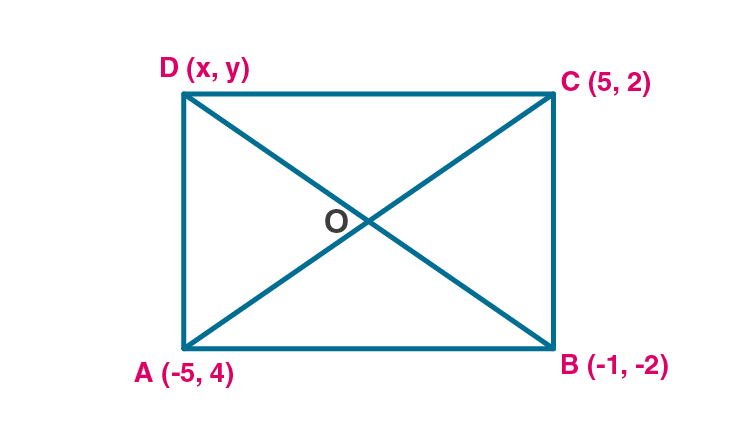Newbie

# Prove that the points A ( – 5, 4), B ( – 1, – 2) and C (5, 2) are the vertices of an isosceles right angled triangle. Find the co-ordinates of D so that ABCD is a square.

• 0

An Important Question of class 10 Based on Section Formula Chapter of M.L Aggarwal for ICSE BOARD.
Here you have to Prove that the three points given are the vertices of an isosceles right angled triangle.
And find the coordinates asked in the question.
This is the Question Number 26, Exercise 11 of M.L Aggarwal.

Share

1.Given points are A(-5,4), B(-1,-2) and C(5,2) are given.

Since these are vertices of an isosceles triangle ABC then AB = BC.

By distance formula, d(AB) = √[(x2-x1)2+(y2-y1)2]

Here x1 = -5, y1 = 4

x2 = -1, y2 = -2

d(AB) = √[(-1-(-5))2+(-2-4)2]

d(AB) = √[(4)2+(6)2]

d(AB) = √(16+36)

d(AB) = √52 …(i)

By distance formula, d(BC) = √[(x2-x1)2+(y2-y1)2]

Here x1 = -1, y1 = -2

x2 = 5, y2 = 2

d(BC) = √[(5-(-1))2+(2-(-2))2]

d(BC) = √[(6)2+(4)2]

d(BC) = √(36+16)

d(BC) = √52 …(ii)

From (i) and (ii) AB = BC

So given points are the vertices of isosceles triangle.

By distance formula, d(AC) = √[(x2-x1)2+(y2-y1)2]

Here x1 = -5, y1 = 4

x2 = 5, y2 = 2

d(AC) = √[(5-(-5))2+(2-4)2]

d(AC) = √[(10)2+(-2)2]

d(AC) = √(100+4)

d(AC) = √104 ….(iii)

Apply Pythagoras theorem to triangle ABC

AB2+BC2 = (√52)2+(√52)2

= 52+52

= 104 ….(iv)

AC2 = (√104)2 = 104…(v)

From (iv) and (v) we got

AB2+BC2 = AC2

So Pythagoras theorem is satisfied.

So the triangle is an isosceles right angled triangle.

Hence proved.

If ABCD is a square, let the diagonals meet at O.

Diagonals of a square bisect each other. So, O is the midpoint of AC and BD.

Consider A-O-C

x= -5, y1 = 4

x= 5, y2 = 2

By midpoint formula, x = (x1+x2)/2

x = (-5+5)/2 = 0/2 = 0

By midpoint formula, y = (y1+y2)/2

y = (4+2)/2 = 6/2 = 3

So co-ordinate of O is (0,3).

Consider B-O-D

Let co-ordinate of D be (x2,y2)

x1 = -1, y1 = -2

x = 0, y = 3

By midpoint formula, x = (x1+x2)/2

0 = (-1+x2)/2

-1+x2 = 0

x2 = 1

By midpoint formula, y = (x1+x2)/2

3 = (-2+y2)/2

6 = -2+y2

y2 = 6+2 = 8

Hence the co-ordinates of the point D are (1,8).

• 0